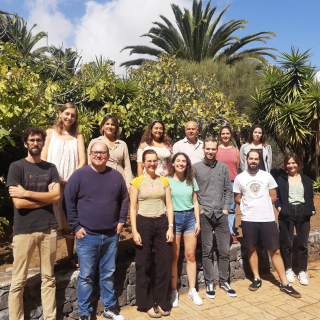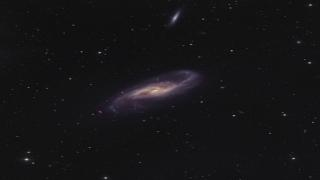# The number of globular clusters around the iconic UDG DF44 is as expected for dwarf galaxies

Saifollahi, Teymoor; Trujillo, Ignacio; Beasley, Michael A.; Peletier, Reynier F.; Knapen, Johan H.
Referencia bibliográfica

Monthly Notices of the Royal Astronomical Society

Fecha de publicación:
4
2021
Descripción
There is a growing consensus that the vast majority of ultradiffuse galaxies (UDGs) are dwarf galaxies. However, there remain a few UDGs that seem to be special in terms of their globular cluster (GC) systems. In particular, according to some authors, certain UDGs exhibit large GC populations when compared to expectations from their stellar (or total) mass. Among these special UDGs, DF44 in the Coma cluster is one of the better-known examples. DF44 has been claimed to have a relatively high number of GCs, $N_{\mathrm{ GC}}=74^{+18}_{-18}$ , for a stellar mass of only $3\times 10^8\, \mathrm{ M}_{ \odot}$ which would indicate a much larger dark halo mass than dwarfs of similar stellar mass. In this paper, we revisit this number and, contrary to previous results, find $N_{\mathrm{ GC}}=21^{+7}_{-9}$ assuming that the distribution of the GCs follows the same geometry as the galaxy. If we assume that the GCs around DF44 are distributed in a (projected) circularly symmetric way and, if we use a less strict criterion for the selection of the GCs, we find $N_{\mathrm{ GC}}=18^{+23}_{-12}$ . Making use of the MGC-Mhalo relation, this number of GCs suggests a dark matter halo mass of $M_{\mathrm{ halo}}=1.1^{+0.4}_{-0.5} \times 10^{11} \mathrm{ M}_{\odot}$ , a value which is consistent with the expected total mass for DF44 based on its velocity dispersion, $\sigma =33^{+3}_{-3}$ km s-1. We conclude that the number of GCs around DF44 is as expected for regular dwarf galaxies of similar stellar mass and DF44 is not extraordinary in this respect.Huellas de la Formación de las Galaxias: Poblaciones estelares, Dinámica y Morfología

Bienvenida a la página web del grupo de investigación Traces of Galaxy Formation. Somos un grupo de investigación amplio, diverso y muy activo cuyo objetivo principal es entender la formación de galaxias en el Universo de una manera lo más completa posible. Con el estudio detellado de las poblaciones estelares como bandera, estamos constantemente

Ignacio
Martín NavarroLas Galaxias Espirales: Evolución y Consecuencias

Nuestro grupo pequeño esta bien conocido y respetado internacionalmente por nuestro trabajo inovativo e importante en varios aspectos de la estructura y la evolución de las galaxias espirales cercanas. Usamos principalmente observaciones en varias longitudes de onda, explotando las sinergías que nos permiten responder a las cuestiones más

Johan Hendrik
Knapen Koelstra